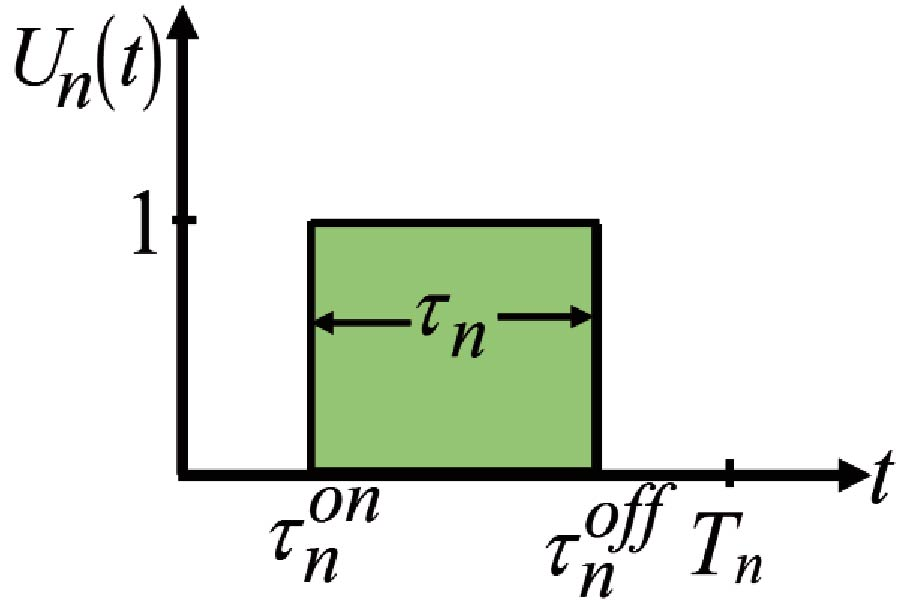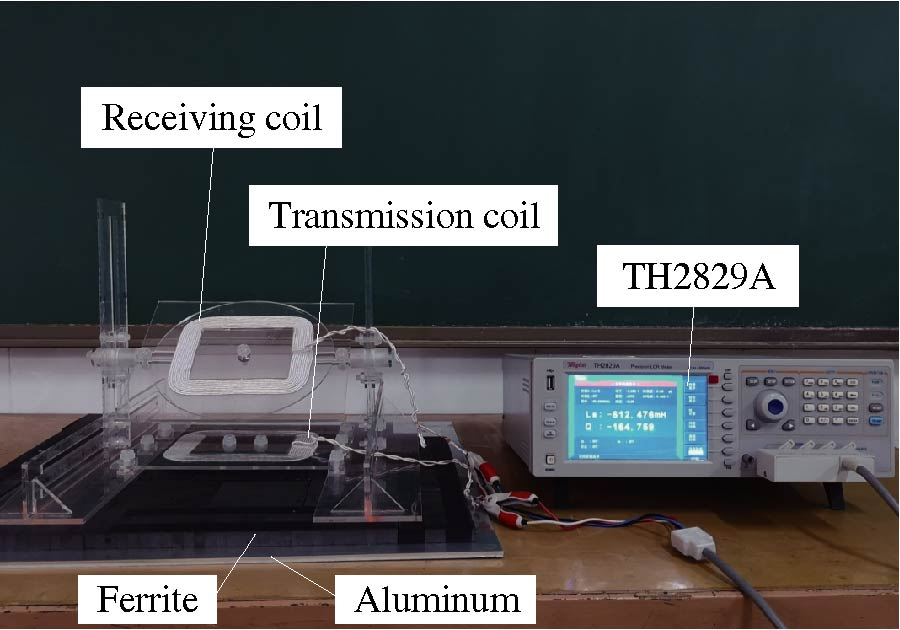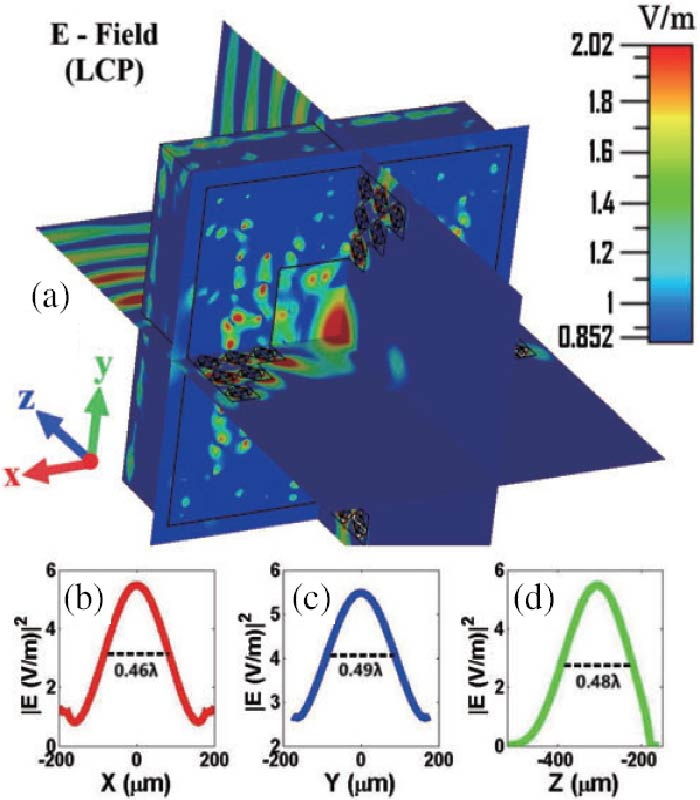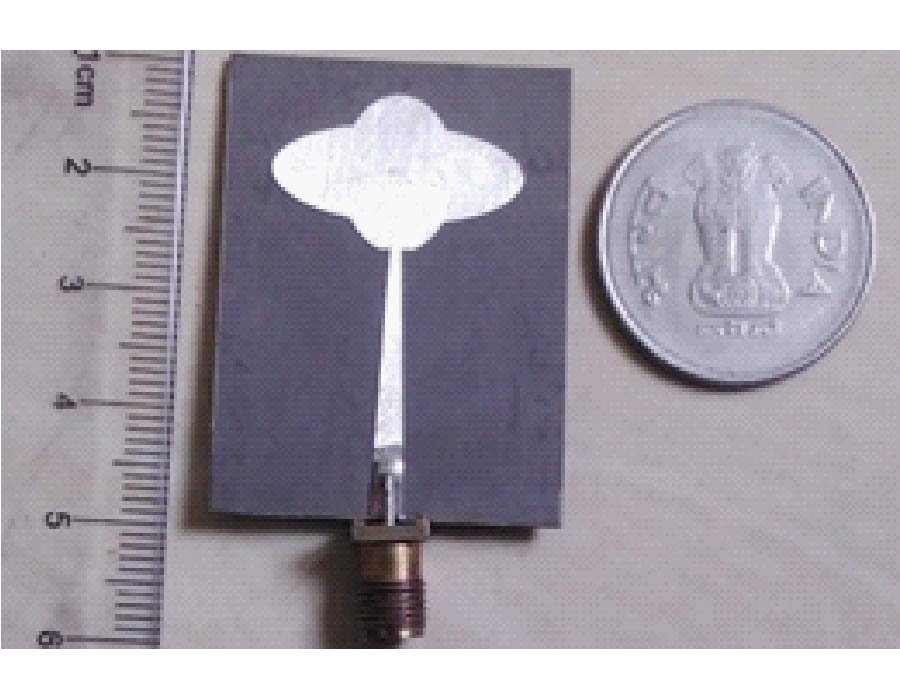Vol. 98
Latest Volume
All Volumes
All Issues
2023-01-27
PIER B
Vol. 98, 59-75, 2023
Efficient Computation of Sideband Power Losses in Pulse-Shifted Non-Uniform Time-Modulated Array with Arbitrary Element Pattern
Sujoy Mandal Sujit Kumar Mandal
This paper presents the mathematical formulation for the generalized closed-form expressions to calculate sideband power (PSR) of a nonuniform period time modulated array (NTMA) antenna with volumetric geometry by using pulse shifting strategy. For the arbitrary array geometry, the generalized expression of PSR is obtained by considering the universal omnidirectional element pattern in the form sinaθ|cosθ|b, a > -1, b > -1/2. Then, corresponding to different array structures such as linear, planar, and volumetric ones, the derived expression is simplified for different element patterns with possible combination of `a' and `b'. Through representative numerical results it is demonstrated that the obtained simplified expressions without hypergeometric function are useful to accurately calculate the amount of power losses due to sideband radiations with significantly less time than the conventional numerical integration (NI) method.2023-01-04
PIER B
Vol. 98, 39-57, 2023
Coupling Coefficient Calculation of Arbitrarily Positioned Rectangular Coils with Double Magnetic Shielding in Wireless Power Transfer Systems
Zhongqi Li Zhongbang Chen Jing Li Huadong Liu Qing Huang
Coupling coefficient is a key parameter for the coil design of wireless power transfer (WPT) systems. The accurate calculation of coupling coefficient is an important theoretical basis for optimizing the coil structure and improving the transmission efficiency in WPT systems. In this paper, the magnetic flux density distribution of rectangular spiral coils with double magnetic shielding is studied, and an analytical model of coupling coefficient between arbitrarily positioned rectangular spiral coils is established. First, the incident magnetic flux density is obtained based on the dual Fourier transformation and the relationship between the magnetic flux density and magnetic vector potential. Second, the reflected magnetic flux density in the region of the receiving coil is solved by using Poisson's equation, Laplace's equation and boundary conditions. Finally, the formula for the coupling coefficient between rectangular spiral coils is derived by the spatial frame transformation method and the integral method. The calculation results agree well with the finite element simulation value and experimental measurements, which verifies the correctness of the calculation formula of the coupling coefficient.2022-12-21
PIER B
Vol. 98, 21-37, 2023